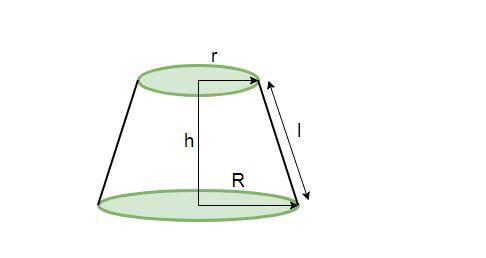Courses
Courses for Kids
Free study material
Free LIVE classes
MoreLIVE
Join Vedantu’s FREE Mastercalss

# Find the curved surface area of the frustum with slant height of 4 cm and the perimeters (circumference) of its circular ends are 18 cm and 6 cm.Verified
360.9k+ views
Hint: We need to know the formula of frustum of cone for solving the above problem. The curved surface area of frustum of cone is given by $\pi (r+R)l$ (as shown in the figure below). Here, r and R are the radii of two circular faces of the frustum. Also, l is the slant height of the frustum.From the question, the perimeters of the circular ends are 18 cm and 6 cm. We know that the formula of the perimeter of circular ends is $2\pi r$(where r is the radius of the circular end). Utilizing this formula-
\begin{align} & 2\pi r=6 \\ & r=\dfrac{3}{\pi } \\ \end{align}
Similarly, for larger circular end,
\begin{align} & 2\pi R=18 \\ & R=\dfrac{9}{\pi } \\ \end{align}
Now, we use the formula given in the hint,
Curved surface area of frustum = $\pi (r+R)l$
Now, we put the values of r and R, we calculated in this equation
$=\pi \left( \dfrac{3}{\pi }+\dfrac{9}{\pi } \right)l$
Further, the value of slant height (that is l) is also given as 4 cm. Thus, substituting this value and then solving-
\begin{align} & =\pi \left( \dfrac{3}{\pi }+\dfrac{9}{\pi } \right)4 \\ & =\pi \left( \dfrac{3+9}{\pi } \right)4 \\ & =\pi \left( \dfrac{12}{\pi } \right)4 \\ & =48 \\ \end{align}

Thus, the curved surface area of the given frustum is $48\text{ c}{{\text{m}}^{2}}$. (The units are $\text{c}{{\text{m}}^{2}}$ because all the units of radii and slant height are in cm)

Note: While solving the problem, keep the values of r and R in terms of $\pi$. This way while calculating the curved surface area, we can cancel the $\pi$ terms from the numerator and the denominator avoiding huge calculations. A common mistake in these types of problems is the inability to understand the difference between total surface area and curved surface area. One should remember that curved surface area is the area of the solid shape excluding the top and bottom cross section areas. The common mistake made in these problems is forgetting to put the units of curved surface area (in this case, $\text{c}{{\text{m}}^{2}}$). Always remember to write the final answer with units.
Last updated date: 21st Sep 2023
Total views: 360.9k
Views today: 3.60k